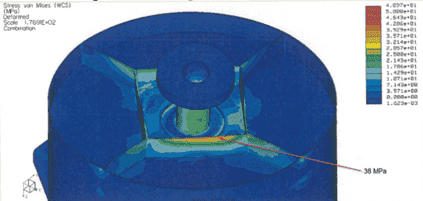# How to calculate the maximum stress in a rib of a complex construction

Ham94
TL;DR Summary
How to calculate a complex construction for the maximum stress or stress in the stiffenerconstruction? there are forces and momentums in the x,y,z, direction. They work in the most worst direction. I don't know how to handle this subject.
A Construction is stiffened by a bottemplate with welded ribs. A flange welded to the inletpipe is bolted at the botomplate. I want to simulate the current situation. Therfore I need a handcalculation of the stresses in the ribs of the constuction. The forces and moments are working in the worst case direction.
fx=6kN
fy=6kN
fz=6kN
Mx=6kNm
My=6kNm
Mz=6kNmunderside
Casingdiameter =900 mm
Casingthickness=5 mm
Botemplatethickness=5 mm
squareribs= 400*400
ribthickness=5 mm
height rib= 120 mm
length of ribs form square to circular casing= 4* 162 mm (angle from center 90=°, t=5 mm)
hole bottomplate= 250 mm
flangediamater= 350 mm

I don't know how to handle this situation. I want to calculate the maximum stresses in the ribs OR the stresses in a rib at one point.
Is there a possability to calculate without a inertia moment?
I have tried to calculatie the inertia moment of te ribs, but by second thought it is difficult because of the integrals and the positions of the ribs.
Maybe my thought is even harder than the solution.

Last edited: